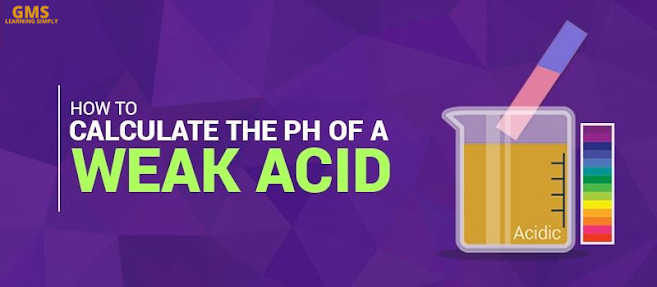# How to Calculate the pH of a Weak AcidCalculate the pH of a Weak Acid with the help of an Example  – Chemistry

The pH scale is what we use to measure the level of acidity and basicity of a substance. It is approximately the negative of the base 10 logarithm of hydrogen ion (H+) activity. According to their pH values, we have categorised aqueous solutions into two, namely acids and bases.

Acids are solutions with a pH value less than 7 whereas bases are solutions with a pH value greater than 7. Pure water is neutral at 25 °C and has the standard neutral pH value of 7. The pH value of a solution can go lower than 0 and greater than 14 in the case of really strong acids and bases.

## Calculations of pH

The calculation of pH of acids and bases are done on the basis of chemical speciation calculation. The nature of the solution often decides the level of sophistication required to calculate its pH value. In our case, let us see how to calculate the pH of a weak acid.

## pH of a weak acid

Since weak acids don’t completely dissociate in water, calculating the pH value is difficult. Let us look at how to calculate the pH value of a weak acid with the help of an example.

Example:

Find the pH of benzoic acid solution?

Given:

For the given sample of 0.01 M of benzoic acid,

Ka = 6.5 x 10-5In the above equation,

[HB] = concentration of undissociated acid molecules [B] = concentration of base [H+] = concentration of H+ ions

In this particular case, the concentration of H+ ions = [ C6H5COO- ]

For every C6H5COO-, benzoic acid gives out one H+ ion.

Let Y be the initial concentration and c be the concentration of H+, then we can say that [H B] = Y – c( Y – c ) Ka = c2

c2 = Y Ka – c Ka

c2 + Kac – YKa = 0

Solving the above equation using quadratic equations we getWhile solving we will get two solutions, but then we consider the feasible value as c cannot be negative.

Ka = 6.5 x 10-5

γ = 0.01 Mc = 7.7 x 10-4

To find the pH we use the equation,

pH = – log [H+]

pH = – log [c]

pH = – log [ 7.7 x 10-4]

pH = – [-3.11]

pH = 3.11

Thus we can say that we calculated the pH of 0.01 M benzoic acid solution and the pH was found to be 3.11 which is acidic.

•I bring to you the BEST for students of Class 9th - 12th. I (Balkishan Agrawal) aim at providing complete preparation for CBSE Board Exams (Maths) along with several other competitive examinations like NTSE, NSO, NSEJS, PRMO, etc. & Maths Reasoning, Vedic Maths at the school level. Wishing you great learning in all aspects of your life!

error: Content is protected !!